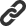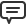# Blog

All Blog Posts  |  Next Post  |  Previous Post

#The Exit Procedure

Bookmarks:

#### Monday, February 05, 2018All Pascal programmers know the `Exit()` procedure since the early versions of the compilers. But do they know how to use it correctly?

The `Exit()` procedure is used when we want to exit of running scope. That scope could be a function, procedure, method, or even the program itself.

Let's say that a console program calls a procedure named `Execute`:

```    procedure Execute;
begin
Writeln('1. Passing on this line...');
Exit;
Writeln('2. It will not pass here');
end;
```

In the example above, only the information from the first `Writeln` will be shown on the console.

When exiting a scope, the program immediately returns to the previous scope (another function/procedure/method or the program itself). The only exception to this rule is when there are `try-finally` blocks. If `Exit ()` is called within a `try-finally` block, the compiler will execute the code inside the `finally-end` before it exits the scope.

Here is another example:

```    procedure Execute;
begin
try
Writeln('1. Passing on this line...');
Exit;
finally
Writeln('2. I am still here!');
end;
Writeln('3. It will not pass here');
end;
```

Texts #1 and #2 will be shown on the console. Even though `Exit()` was called before the text #2 was printed, the code still runs because of `try-finally`.

Another example of using `Exit()` is when we do validations. If a validation or checking does not return true, we use `Exit()` to stop execution of the current scope.

Suppose that we want to add two integer numbers, but we only want integers bigger than zero:

```    function Sum(A, B: Integer): string;
begin
Result := 'Invalid result';
if (A < 0) or (B < 0) then
Exit;
Result := Format('The result is %d', [A + B]);
end;
```

In the above example, the return of `Sum` function is initialized with an invalid value and then there is a validation to know if the values are less than `0`. If the test fails, the program will return to the prior scope to calling `Sum` function with the invalid result. But if the test does not fail, the function result will be the sum of A and B.

There are those who are adept at structured programming and prefer do not "break" the program execution with an "early exit", which means they do not use `Exit()` because they believe the code would be simpler.

So, let's rewrite the previous example:

```    function Sum(A, B: Integer): string;
begin
if (A > 0) and (B > 0) then
Result := Format('The result is %d', [A + B]);
else
Result := 'Invalid result';
end;
```

Looks simpler? Well, in this example I would say yes. But for examples with more conditionals, I'd say no (let's see this below).

What if we wanted to tell the user that their data is not correct?

```    function Sum(A, B: Integer): string;
begin
Result := 'Invalid result';
if (A > 0) then
begin
if (B > 0) then
Result := Format('The result is %d', [A + B]);
else
Writeln('B should be greater than zero');
end
else
Writeln('A should be greater than zero');
end;
```

In this example, we do not use `Exit()` and I think the code is quite confusing. The tests are "separated" from the warning return to the user (`Writeln`).

Kent Beck, Martin Fowler stated categorically that "one exit point is really not a useful rule. Clarity is the key principle: If the method is clearer with one exit point, use one exit point, otherwise don't".

So, let's rewrite the previous example using `Exit()`:

```    function Sum(A, B: Integer): string;
begin
Result := 'Invalid result';
if (A < 0) then
begin
Writeln('A should be greater than zero');
Exit;
end;
if (B < 0) then
begin
Writeln('B should be greater than zero');
Exit;
end;
Result := Format('The result is %d', [A + B]);
end;
```

The code got a little bigger, it's true, but the tests and warnings for the user got simpler, in my opinion. You do not have to follow all nested `if-else's`. For each test that fails, the warning is just below and the scope will be aborted with the use of `Exit`. If all tests do not fail, the function will return the sum of A and B.

In Delphi, as of 2009 version, `Exit()` procedure has gained an improvement: `Exit()` can have a parameter specifying a result. The parameter must be of the same type as the result of the function.

The FPC also has the same definition, but I do not know who implemented this new feature first.

Again, let's rewrite the previous example:

```    function Sum(A, B: Integer): string;
begin
if (A < 0) then
Exit('A should be greater than zero');
if (B < 0) then
Exit('B should be greater than zero');
Result := Format('The result is %d', [A + B]);
end;
```

Simple and clean.

`Exit()` can be given any type of return, even instances of Interfaces. Using this parameter, it is as if we get the same behavior as the `return` reserved word in Java. However, `Exit()` together with `Result`, gives us even more possibilities to return from functions.

See you.

Marcos Douglas B. Santos

Bookmarks:1. Monday, February 05, 2018 at 7:28:14 PM

I can comment just on one thing: "exit(value)" is part of FreePascal 1.0 release (which was launched many years before Delphi 2009).

FPC though copied over time many features from Delphi, but FPC was the source on this one

Ciprian K2. Tuesday, February 06, 2018 at 11:53:53 AM

Ciprian,
I didn''t know that. Thanks for this information.

Marcos Douglas3. Tuesday, February 06, 2018 at 9:04:03 PM

Congratulations Marcos Douglas, I hope to see new articles soon.
Thank you.

Manoel Pedro G. M. Jr